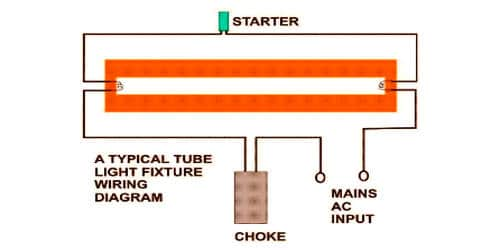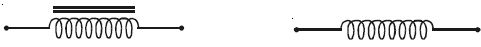# Describe Working Procedure of Choke Coil

A choke coil is an inductance coil of very small resistance used for controlling current in an a.c. circuit. It is a part used in the electrical circuits to allow DC current to flow through while blocking AC current from passing. If resistance is used to control current, there is a wastage of power due to the Joule heating effect in the resistance. The low electrical resistance allows DC and AC to pass with little power loss but it can limit the amount of AC passing through it due to its reactance.

Working Procedure of Choke Coil:

A choke coil is an inductance coil of very small resistance used for controlling current in an a.c. circuit. If resistance is used to control current, there is a wastage of power due to the Joule heating effect in the resistance.

The inductive reactance offered by the coil is given by XL = ωL

In the case of an ideal inductor the current lags behind the emf by a phase angle π/2.

∴ The average power consumed by the choke coil over a complete cycle is

Pav = Erms Irms cos π/2 = 0

However, in practice, a choke coil of inductance L possesses a small resistance r. Hence it may be treated as a series combination of an inductor and small resistance r. In that case, the average power consumed by the choke coil over a complete cycle is

Pav = Erms Irms cos φ

Pav = Erms Irms [r/( r 22 L2)] … … (1)

where [r/( r 22 L2)] is the power factor. From equation (1) the value of average power dissipated works out to be much smaller than the power loss I2R in a resistance R.Fig: an A.F Choke                             Fig: b R.F. Choke

Chokes used in low-frequency a.c. Circuits have an iron core so that the inductance may be high. These chokes are known as audio – frequency (A.F) chokes. For radio frequencies, air chokes are used since a low inductance is sufficient. These are called radiofrequency (R. F) or high frequency (H.F) chokes and are used in wireless receiver circuits (Fig: a and Fig: b).

Choke coils can be commonly seen in fluorescent tubes that work on alternating currents.

The choke coil works because it can act as an inductor. When the current pass through will change as AC currents create a magnetic field in the coil that works against that current. This property, known as inductance, blocks most of the AC current from passing through. As a result, currents that do not change, such as DC currents, can continue through while those that do are blocked by the very magnetic field they created.

At the core of this part is a material used to produce its magnetic field. Coils used to filter higher frequencies may use an air-core or a ferrite core, while lower frequency coils may use an iron core. The material in the core determines the strength of the magnetic field and the inductance rate of the coil.